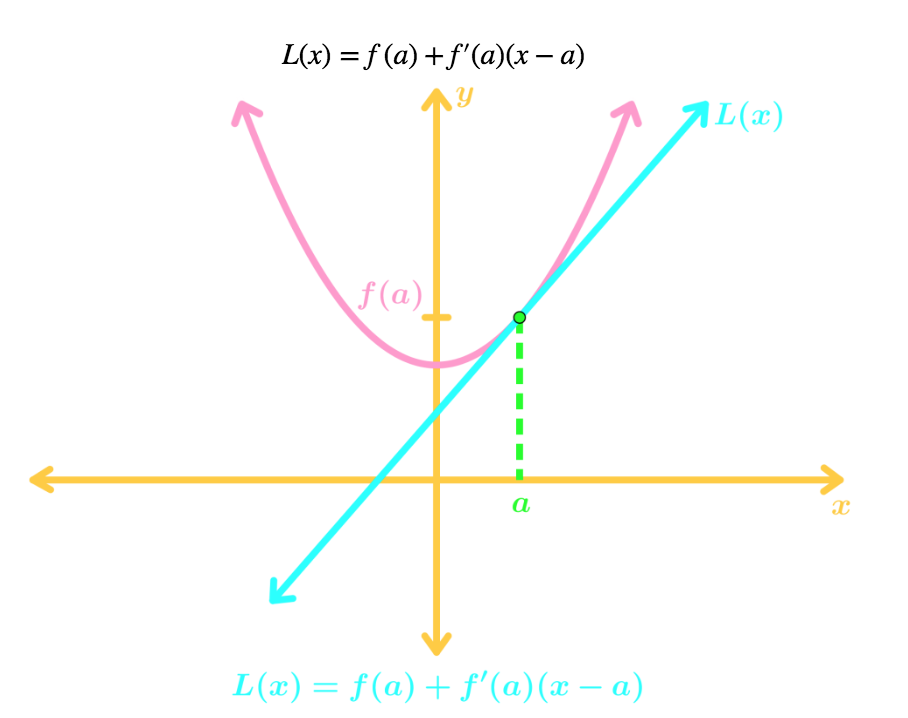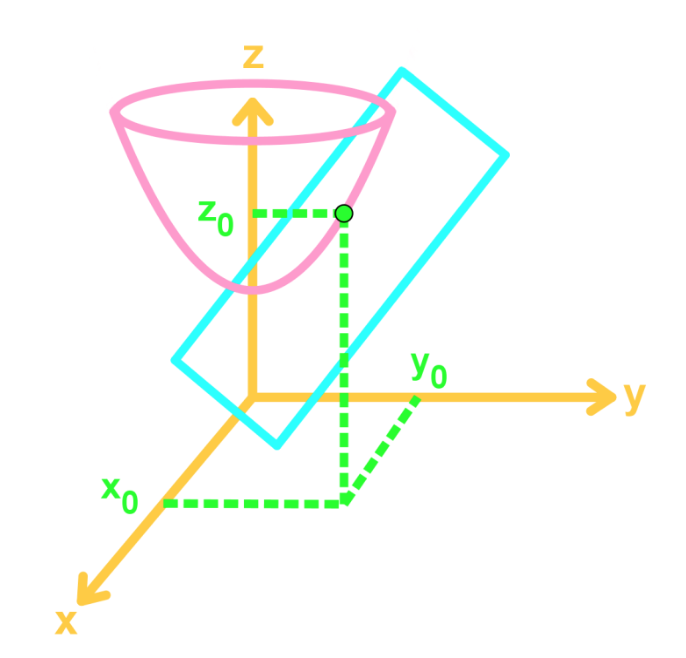# Linear approximations and tangent planes

### Linear approximations and tangent planes

#### Lessons

Notes:

Tangent Planes
Recall that in Calc I, linear approximation is about finding a linear equation tangent to a curve at a point, and using it to estimate values of the curve "near" that point. The equation of the tangent line at point $a$ would be:Calc III is similar, but now it is in 3D. Instead of 2D curves, we have 3D surfaces. Instead of tangent lines, we have tangent planes. The formula for the tangent plane at point $(x_0, y_0)$is:

$z=f(x_0,y_0)+f_x(x_0,y_0)(x-x_0)+f_y(x_0,y_0)(y-y_0)$

Linear Approximation
Just like how we can estimate values of a 2D curve, can also estimate the value of a 3D surface near a point using linear approximation. We say that if we are at the point $(x_0,y_0)$, and we want to approximate a point near it (say $(x,y)$), then

$f(x,y) \approx f(x_0,y_0)+f_x(x_0,y_0)(x-x_0)+f_y(x_0,y_0)(y-y_0)$• Introduction
Linear Approximations & Tangent Planes Overview:
a)
Tangent Planes
• A review of linear approximation
• 2D = tangent lines, 3D = tangent planes
• $z=f(x_0,y_0)+f_x(x_0,y_0)(x-x_0)+f_y(x_0,y_0)(y-y_0)$
• An example

b)
Linear Approximation with Multi-Variable Functions
• Estimating a value of a 3D surface
• Approximation for points "near" $(x_0,y_0)$,
• An example